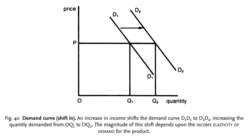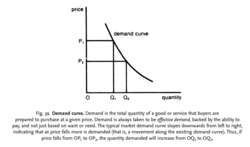# demand curve

(redirected from demand curves)

## demand curve

see EQUILIBRIUM MARKET PRICE.

## demand curve

a line showing the relationship between the PRICE of a PRODUCT or FACTOR OF PRODUCTION and the quantity DEMANDED per time period, as in Fig. 39.

Most demand curves slope downwards because (a) as the price of the product falls, consumers will tend to substitute this (now relatively cheaper) product for others in their purchases; (b) as the price of the product falls, this serves to increase their real income, allowing them to buy more products

(see PRICE EFFECT, INCOME EFFECT, SUBSTITUTION EFFECT). In a small minority of cases, however, products can have an UPWARD-SLOPING CURVE.

The slope of the demand curve reflects the degree of responsiveness of quantity demanded to changes in the product's price. For example, if a large reduction in price results in only a small increase in quantity demanded (as would be the case where the demand curve has a steep slope) then demand is said to be price inelastic (see PRICE-ELASTICITY OF DEMAND).

The demand curve interacts with the SUPPLY CURVE to determine the EQUILIBRIUM MARKET PRICE. See DEMAND FUNCTION, DEMAND CURVE ( SHIFT IN), DIMINISHING MARGINAL UTILITY, MARGINAL REVENUE PRODUCT.Fig. 40 Demand curve (shift in). An increase in income shifts the demand curve D1 D1 to D2 D2, increasing the quantity demanded from OQ1 to OQ2. The magnitude of this shift depends upon the INCOME ELASTICITY OF DEMAND for the product.Fig. 39 Demand curve. Demand is the total quantity of a good or service that buyers are prepared to purchase at a given price. Demand is always taken to be effective demand, backed by the ability to pay, and not just based on want or need. The typical market demand curve slopes downwards from left to right, indicating that as price falls more is demanded (that is, a movement along the existing demand curve). Thus, if price falls from OP1 to OP2, the quantity demanded will increase from OQ1 to OQ2 .

## demand curve (shift in)

a movement of the DEMAND CURVE from one position to another (either left or right) as a result of some economic change other than price. A given demand curve is always drawn on the CETERIS PARIBUS assumption that all the other factors affecting demand (income, tastes, etc.) are held constant. If any of these changes, however, then this will bring about a shift in the demand curve. For example, if income increases, the demand curve will shift to the right, so that more is now demanded at each price than formerly. See Fig. 40 . See also DEMAND FUNCTION, INCOME-ELASTICITY OF DEMAND.
References in periodicals archive ?
Aggregate demand for all consumers is the sum of both their demand curves, which is added horizontally (right).
The rest of the paper is outlined as follows: Section II goes through a brief history of Rothbard (2015 ) and his changing ideas on production theory; Section III juxtaposes Rothbard's analyses of monopoly prices and perfect competition; and Section IV compares Rothbard's analyses of the derivation of factor demand curves.
The obtained results appeared visually similar to demand curves derived from human operant studies and were well accounted for by quantitative models of demand (Hursh et al.
Focusing on the economic behavior of individual economic decision units, the undergraduate textbook examines supply and demand curves, constrained optimization, comparative statics of consumer choice, marginal cost curves, the competitive market model, theories of monopoly, price determination in imperfectly competitive markets, and general equilibrium theory.
The second assumption is that any difference in the price level elasticity of aggregate demand for this paper arises only from either the real balance or interest rate effect, with the corollary being that the price level sensitivity of the balance of trade is assumed equal for aggregate demand curves of different price level elasticities.
The workshop addressed the main factors influencing the supply and demand curves, evolution of pricing mechanisms on international natural gas markets as well as regional perspectives of current and future gas pricing mechanisms.
Taken together, therefore, there is considerable evidence that consumers place a positive premium on animal-welfare attributes, but very little evidence on the differential effects of ads for or against the passage of animal welfare regulations or the welfare effects that result from shifting or rotating demand curves.
Once students have this valuable hands-on learning experience, it appears that giving students the tools to further explore foreign exchange markets is the province of traditional supply and demand curves.
But there is a critical assumption involved in his analysis; to wit: the individual firm is still concerned only with the individual demand curves it faces, and these curves have not shifted.
Oil Drums, oil rigs, and supply and demand curves get stretched, defining an ironic image of "stretching" oil production.
Should some basic wholesale data be included in the guidelines, for instance, information on aggregate supply and demand curves, prices and volumes for each standard traded product and for each market timeframe (forward, day-ahead, intra-day) as well as prices and volumes of the over-the-counter market?
To provide a concrete example, suppose that the labor supply and labor demand curves are given by [Q.

Site: Follow: Share:
Open / Close